numpy operations are usually done element-by-element which requires two arrays to have exactly the same shape:

Example 1
```>>> from numpy import array
>>> a = array([1.0, 2.0, 3.0])
>>> b = array([2.0, 2.0, 2.0])
>>> a * b
array([ 2.,  4.,  6.])
```

numpy’s broadcasting rule relaxes this constraint when the arrays’ shapes meet certain constraints. The simplest broadcasting example occurs when an array and a scalar value are combined in an operation:

Example 2
```>>> from numpy import array
>>> a = array([1.0,2.0,3.0])
>>> b = 2.0
>>> a * b
array([ 2.,  4.,  6.])
```

The result is equivalent to the previous example where `b` was an array. We can think of the scalar `b` being stretched during the arithmetic operation into an array with the same shape as `a`. The new elements in `b`, as shown in Figure 1, are simply copies of the original scalar. The stretching analogy is only conceptual. numpy is smart enough to use the original scalar value without actually making copies so that broadcasting operations are as memory and computationally efficient as possible. Because Example 2 moves less memory, (`b` is a scalar, not an array) around during the multiplication, it is about 10% faster than Example 1 using the standard numpy on Windows 2000 with one million element arrays.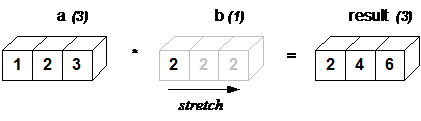Figure 1

In the simplest example of broadcasting, the scalar ``b`` is stretched to become an array of with the same shape as ``a`` so the shapes are compatible for element-by-element multiplication.

The rule governing whether two arrays have compatible shapes for broadcasting can be expressed in a single sentence.

In order to broadcast, the size of the trailing axes for both arrays in an operation must either be the same size or one of them must be one.

If this condition is not met, a `ValueError('frames are not aligned')` exception is thrown indicating that the arrays have incompatible shapes. The size of the result array created by broadcast operations is the maximum size along each dimension from the input arrays. Note that the rule does not say anything about the two arrays needing to have the same number of dimensions. So, for example, if you have a 256 x 256 x 3 array of RGB values, and you want to scale each color in the image by a different value, you can multiply the image by a one-dimensional array with 3 values. Lining up the sizes of the trailing axes of these arrays according to the broadcast rule shows that they are compatible

 Image (3d array) 256 x 256 x 3 Scale (1d array) 3 Result (3d array) 256 x 256 x 3

In the following example, both the `A` and `B` arrays have axes with length one that are expanded to a larger size in a broadcast operation.

 A (4d array) 8 x 1 x 6 x 1 B (3d array) 7 x 1 x 5 Result (4d array) 8 x 7 x 6 x 5

Below, are several code examples and graphical representations that help make the broadcast rule visually obvious. Example 3 adds a one-dimensional array to a two-dimensional array:

Example 3
```>>> from numpy import array
>>> a = array([[ 0.0,  0.0,  0.0],
...            [10.0, 10.0, 10.0],
...            [20.0, 20.0, 20.0],
...            [30.0, 30.0, 30.0]])
>>> b = array([1.0, 2.0, 3.0])
>>> a + b
array([[  1.,   2.,   3.],
[ 11.,  12.,  13.],
[ 21.,  22.,  23.],
[ 31.,  32.,  33.]])
```

As shown in Figure 2, `b` is added to each row of `a`. When `b` is longer than the rows of `a`, as in Figure 3, an exception is raised because of the incompatible shapes.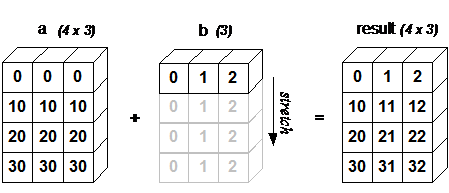Figure 2

A two dimensional array multiplied by a one dimensional array results in broadcasting if number of 1-d array elements matches the number of 2-d array columns.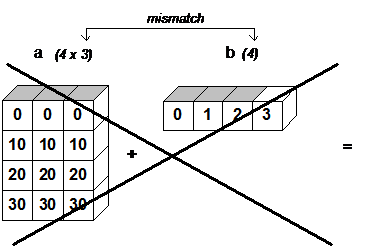Figure 3

When the trailing dimensions of the arrays are unequal, broadcasting fails because it is impossible to align the values in the rows of the 1st array with the elements of the 2nd arrays for element-by-element addition.

Broadcasting provides a convenient way of taking the outer product (or any other outer operation) of two arrays. The following example shows an outer addition operation of two 1-d arrays that produces the same result as Example 3

Example 4
```>>> from numpy import array, newaxis
>>> a = array([0.0, 10.0, 20.0, 30.0])
>>> b = array([1.0, 2.0, 3.0])
>>> a[:,newaxis] + b
array([[  1.,   2.,   3.],
[ 11.,  12.,  13.],
[ 21.,  22.,  23.],
[ 31.,  32.,  33.]])
```

Here the newaxis index operator inserts a new axis into `a`, making it a two-dimensional 4x1 array. Figure 4 illustrates the stretching of both arrays to produce the desired 4x3 output array.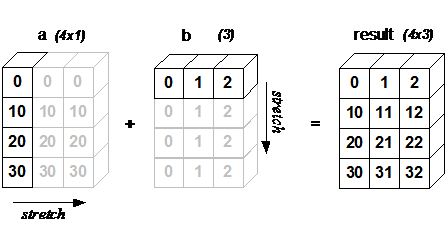Figure 4

In some cases, broadcasting stretches both arrays to form an output array larger than either of the initial arrays.*

## A Practical Example: Vector Quantization.¶

Broadcasting comes up quite often in real world problems. A typical example occurs in the vector quantization (VQ) algorithm used in information theory, classification, and other related areas. The basic operation in VQ [#f0] finds the closest point in a set of points, called codes in VQ jargon, to a given point, called the observation. In the very simple, two-dimensional case shown in Figure 5, the values in observation describe the weight and height of an athlete to be classified. The codes represent different classes of athletes. 2 Finding the closest point requires calculating the distance between observation and each of the codes. The shortest distance provides the best match. In this example, `codes` is the closest class indicating that the athlete is likely a basketball player.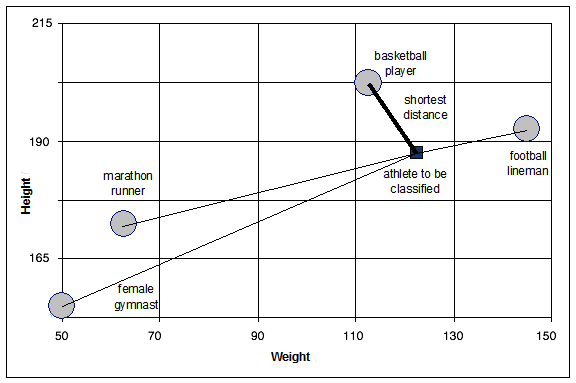Figure 5

The basic operation of vector quantization calculates the distance between an object to be classified, the dark square, and multiple known codes, the gray circles. In this simple case, the codes represent individual classes. More complex cases use multiple codes per class.

Footnotes

1

Vector Quantization J. Makhoul, S. Roucos, and H. Gish, “Vector Quantization in Speech Coding,” Proc. IEEE, vol. 73, pp. 1551-1587, Nov. 1985.

2

In this example, weight has more impact on the distance calculation than height because of the larger values. In practice, it is important to normalize the height and weight, often by their standard deviation across the data set, so that both have equal influence on the distance calculation.

Note

The code to produce the figures is part of the AstroML book